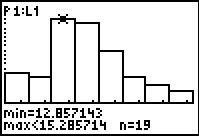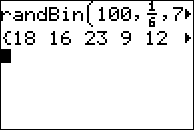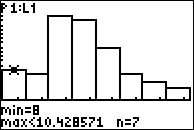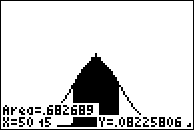# Activities

••• ##### Subject Area

• Math: Statistics: Data Analysis
• Math: Statistics: Normal Distributions

• ##### Author9-12

60 Minutes

• ##### Device
• TI-83 Plus Family
• TI-84 Plus
• TI-84 Plus Silver Edition
•TI-84 Plus C Silver Edition
•TI-84 Plus CE

## What's Normal, Anyway?#### Activity Overview

Students explore normal distribution and several properties. First, simulate a binomial experiment and use a histogram of the data to examine the general shape of a normal curve. They graph a normal distribution given the mean and standard deviation. They see how the graph changes when just the mean or standard deviation changes. Lastly, they further examine normal distributions, describing the percent of data values falling within different standard deviations from the mean.

#### Key Steps

•Students begin the activity by simulating 75 experiments of rolling a die 100 times and recording the number of successes where a success is rolling a "3".

•Students will create a histogram of their data.

•Students will explore the area under the normal curve.